## Is the point (1,5)a solution to the equation y=2x+3?​

Question

Is the point (1,5)a solution to the equation y=2x+3?​

in progress 0
7 months 2021-07-17T10:22:39+00:00 1 Answers 3 views 0

## Answers ( )

1. Step-by-step explanation: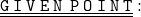• ( 1 , 5 )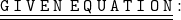• y = 2x + 3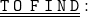• Whether the point ( 1 , 5 ) , a solution to the equation y = 2x + 3 or not.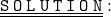Assuming the the value of x and y as 1 and 5 respectively. Now , plug the values of x and y in the given equation & let’s see whether the point ( 1 ,5 ) , solution to the equation y = 2x + 3 or not !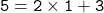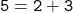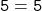Since we got 5 on R.H.S & L.H.S, So yeah! The point ( 1 , 5 ) is a solution to the equation y = 2x + 3.

Hope I helped ! ♡

Have a wonderful day / night ! ツ

#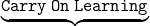!! ✎

▁▁▁▁▁▁▁▁▁▁▁▁▁▁▁▁▁▁▁▁▁▁▁▁▁